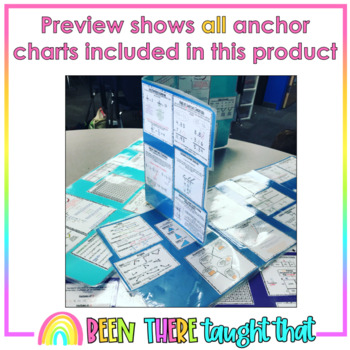# 5th Grade Math Personal Mini Anchor ChartsSubject
Resource Type
File Type

PDF

(1 MB)
Product Rating
Standards
• Product Description
• StandardsNEW

Why did I not think of this sooner?!

All of the great anchor charts I make through out the year I have now made mini for students to use whenever they need them!

Here are over 20 anchor charts covering most of the major 5th grade skills. Print and glue them to file folders to create a personal work space for students. OR print on card stock and laminate and place mini anchor charts on a ring for students to reference when needed.

My students LOVE these and so do my admin team. It is a great way to set students up for success and for them to practice their problem solving skills by using resources's around them.

Anchor Charts Cover the following:

5.OA.2- Order of Operations

5.NBT.1- Multiply/Divide by powers of ten

•Exponents

•Values of Powers of Ten

5.NBT.5 – Multi-Digit Multiplication

5.NBT.7- Add, Subtract, Multiply & Divide Decimals

5.NF.1- Add & Subtract Fractions with unlike Denominators

•Subtract Mixed Numbers with Borrowing

•Greatest Common Factors & Least Common Multiple

•Simplify Fractions

5.NF.4 Multiply Fractions

5.NF.7 Divide Fractions

•Turn Mixed Numbers to Improper Fractions and Improper Fractions to Mixed Numbers

5.MD.1- Metric and Customary Conversions

5.MD.5- Volume

5.G.1- Graph Points on a Coordinate Plane

OTHER:

Multiplication Chart

Place Value Chart from Hundred Million to Thousandths

Factors & Prime/Composite Numbers

Long Division

Classify two-dimensional figures in a hierarchy based on properties.
Understand that attributes belonging to a category of two-dimensional figures also belong to all subcategories of that category. For example, all rectangles have four right angles and squares are rectangles, so all squares have four right angles.
Use a pair of perpendicular number lines, called axes, to define a coordinate system, with the intersection of the lines (the origin) arranged to coincide with the 0 on each line and a given point in the plane located by using an ordered pair of numbers, called its coordinates. Understand that the first number indicates how far to travel from the origin in the direction of one axis, and the second number indicates how far to travel in the direction of the second axis, with the convention that the names of the two axes and the coordinates correspond (e.g., 𝘹-axis and 𝘹-coordinate, 𝘺-axis and 𝘺-coordinate).
Apply and extend previous understandings of division to divide unit fractions by whole numbers and whole numbers by unit fractions.
Apply and extend previous understandings of multiplication to multiply a fraction or whole number by a fraction.
Total Pages
N/A
N/A
Teaching Duration
N/A
Report this Resource to TpT
Reported resources will be reviewed by our team. Report this resource to let us know if this resource violates TpT’s content guidelines.